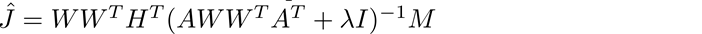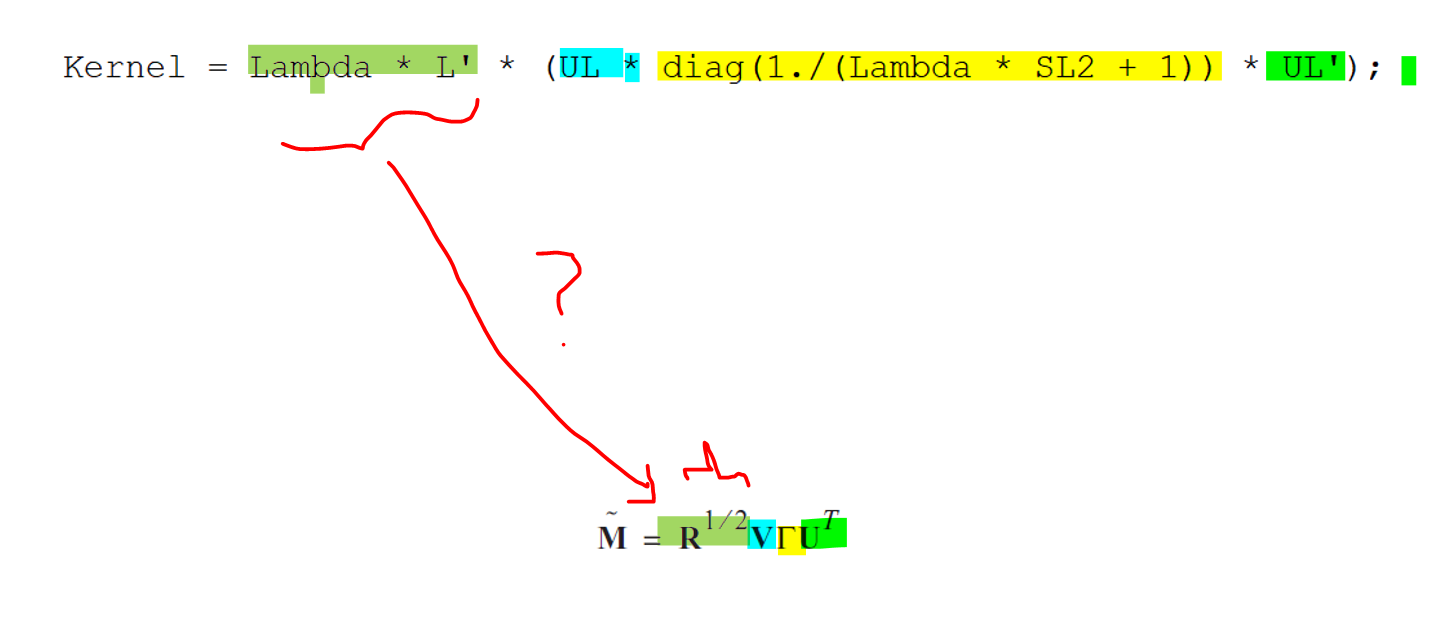# Understanding Source Localization of bst_inverse_linear

Hello,
I am trying very hard to understand the computational side of the inverse problem, in this case, MNE.
I have attached an image from the code.
Firstly, I just want a confirmation for the following:

1. The kernel is the Estimate of the dipoles or the Source which in Eletromagnetic Brain Mapping article is called S^T?

2. L being Lead Field Matrix is composed of Head model which has three components: Head.Gain, Grid.Location and Grid.Orientation. In the case of Fixed orientation, Grid.Location in the matrix of vertices (observed from the forward model) and Head.Gain is a matrix initialized by the **MEASUREMENT? **, This means that it is more correct to say L is A^+ M, since M (measurement) is projected on the Lead field matrix already.

Secondly, I have the following questions:

1. Why are we performing singular value decomposition of the lead field matrix?
2. How can I see the single time point (Dipole Matrix).
3. Which function should I refer to, to understand how the dipoles are projected to the head model for 3D Surface Demonstration?

I am sorry if my questions are more mathematical and technical rather than the performance of the code itself. I am doing my MASc on inverse problem, spent the whole first year on understanding the mathematical concepts behind all methods, now I am stuck on the computational side, I can't get my head around the understanding of how to code the mathematics and the cost function. (If there is any other article you suggest other than Electromagnetic Image Mapping please let me know

With Warm Regards
Younes

image|690x137

Hi Younes:

The SVD indicates the conditioning of the lead field matrix: if the spectrum of singular values is very wide (smaller singular values being several orders fo magnitudes smaller than the largest ones), the matrix is said to be ill conditioned mathematically, which is always the case with MEG and EEG. Computing the SVD spectrum helps determining how much regularization to apply to produce a reasonable (stable) minimum norm estimate of source amplitudes.

I am not sure what you mean, sorry. The result of the MNE is the production of a matrix called the imaging kernel, which is nsources x nsensors. It is a regularized version of the inverse of the gain matrix. If you want to extract the ith source time series, just multiply the ith row of the imaging kernel with with the sensor data array.

Sorry again: I am not sure what you are referring to exactly here. Can you specify a bit more?

1 Like

Hello Dr.Sylvain,
thank you so much for your reply.
I am sorry that my questions were not clear, maybe it is because I have not understood the coding right, to confirm that I would like to ask the following :

1. is the observed Lead Field matrix "L" = multiplication of the noisy data (M) by the head model (grid points) (in matrix gain function) and then whitened and weighted?

2. How is the Kernel equation : Kernel = Lambda * L' * (UL * diag(1./(Lambda * SL2 + 1)) * UL') drived?

3. My general issue is that I can't connect the mathematic derivation of the paper directly with the coding, so I am trying to understand what has happened in the coding:
issame as Kernel = Lambda * L' * (UL * diag(1./(Lambda * SL2 + 1)) * UL'); ?

4. Assuming that we have the kernel image, what function has been used to illustrate the sources on the head surface? (Demonstration Function? )

As always thank you so much for your time

Best
Younes

@Sylvain
I just want to make sure you have recieved notifications for my question above

The lead field matrix is another name for the gain matrix produced by the forward model.

It is a computationally optimized version of the MNE equation you are quoting in #3. @jcmosher can tell you more about how it is derived more specifically.

The kernel is not an image, it is a matrix operator applied to the sensor data to produce an estimate of source time series at every cortical location. It is the large matrix multiplication to the left of M (the sensor data) in the equation in your point #3.

Hope this clarifies.

1 Like

The implementation of the min-norm estimate in Brainstorm is identical to that of MNE, which is nicely detailed in their pdf manual from p. 121.

Hope this useful.

2 Likes

Dear Dr.Sylvain
Thank you so much for the reply and the manual that you sent.
I was wondering, is there any specific research paper focusing on why do we need the regularization parameter or the " + lambda |fs| " function? Is it because of the existence of the noise or because the modeling is not completely correct? I understand that adding the regularization parameter makes the solution more sparse and helps with not getting stuck in local minima while performing L2 Norm, but I was wondering, what is the mathematical problem with the Linear MNE that forces us to use regularization parameters, covariance, and lambda?

Thank you
Best

Hi Younes:
I have a similar problem with you,as show in
https://forum.bic.mni.mcgill.ca/t/brainstorm-tutorial-question/1246/2
The Brainstorm software provided wonderful tutorials for beginners, while, beautiful yet incomplete, there are not enough mathematical formulas for understanding it’s codes.
If you find papers corresponding to ‘bst_inverse_linear’, please share with me.
With Warm Regards
Ming

Regularization is indeed necessary because of the ill-posed nature of the MEG inverse problem: 1) there is no unique solution and 2) solutions are not numerically stable in presence of noise and limited numerical precision.
Regularization is a very generic solution to these issues. MN is one way to obtain a unique, stable solution.

1 Like

Hello Dr.Sylvain
I went through MNE-Manual p.121 to understand the computational aspect of sLORETA in Brainstorm, and I have the following questions

1. I was trying to match the equation provided be MNE and the MATLAB code for Brainstorm MNE and I saw the following:
The only part that I cant understand is the DARK green highlight.Would you please elaborate on that?
Based on MNE R' should be Source co variance, how is that equal to \lambda * L' ?

2. I also read Electromagnetic Brain Mapping papaer and tried to match the given equation there to the MNE equation and Say the following:Is the equation provided in Electromagnetic BRAIN MAPPING assuming WHITENED Kernel (third equation) or pre-whitenning equation? If its whitened, why it has lambda parameter while MNE whitened doesn't have lambda?

Thank you for all your helps!

Best

The idea behind whitening is that it produces a generic form of regularized estimator. Before whitening, the noise covariance statistics are explicitly included in the kernel as C. After whitening, the kernel form assumes independent and identically distributed noise statistics (the I matrix in the kernel 3rd equation above). Our paper assumed, for simplicity, this iid hypothesis (although I think I remember we mentioned that noise covariance statistics could be accounted for in principle in forming the regularized imaging kernel).

1 Like

@unes1111 you may be interested in reading our paper on this:

hope this helps
Alex

1 Like

Hello!
My question may repeat topics discussed here.
But could you explain or give an article with a detailed explanation:
Why do we multiply by raw MEG data when we make an inverse operator MNE or sLORETA?
I know that the notion Regularization plays the crucial role here, but it’s not clear for me how it works.

You may find these references helpful:

Updated link to the MNE manual:

Hi,
I am also trying to understand this part:

and why, in the script, lambda is computed as Lambda = SNR^2/mean(SL.^2) (with SL=sqrt(SL2) ) whereas in MNE manual lambda is approximated as lambda^2=1/SNR.
Any explanation will be very heplful, please.